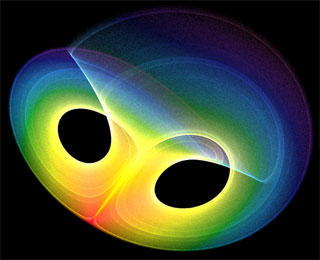# Chaos theory and butterfly effect

## Chaos and randomnessAutomatic translation Category: Earth
Updated November 25, 2013

The world around us often seems unpredictable, disordered, hazardous and chaotic. A chaotic system is a simple or complex system, sensitive to initial conditions and which has a repetitive character, a strong recurrence. A small perturbation can cause instability or unpredictable gigantic long-term imbalance. Thus, simple plan can give rise to complex phenomena. A chaotic system is the reverse of a perfectly regular system. In celestial mechanics, the motions of the planets around the Sun have long seem to belong to a regular system. Since the great astronomical revolution, Nicolas Copernicus (1473-1543) imposes the simple idea that the planets revolve around the Sun. Johannes Kepler (1571-1630) can then calculate with high accuracy, the trajectories of planets around the Sun.
Then Isaac Newton (1642-1727) explains all celestial movements with one simple law, the law of gravitation. The matter attracts matter from the masses involved, and inversely to the square of their distance. This allows for precise calculations and above forecasts. With this simple law all celestial movements are explained. However mismatches exist between astronomical observations and calculations and yet the mathematical laws of gravitation theory is accurate. This comes from the fact that the laws work well in a two celestial objects system but all celestial objects undergo gravitational influence of others. These are these small fluctuations, turbulence, these gravitational perturbations that eventually force the system instability.
Chaos theory has emerged with the computer in the 1970s, computers allowed immediate visualization of complex dynamic systems like the solar system. It is precisely the study of motion of three bodies in gravitational interaction, like that of the Sun, Earth and Moon, which is the origin of chaos theory. The study of the problem of three bodies isolated from the rest of the universe, has allowed to know the stability of the solar system over the long term. The scientists wanted to know the risk of a collision with another body or risks of a body to be ejected from the Solar System. Pierre-Simon Laplace (1749-1827) believed in the stability of the system but the French mathematician Henri Poincaré (1854-1912), a century later, discover the hidden potential chaos in the equations of the theory of dynamical systems.

Poincaré simplifies calculations by studying a solar system containing only three bodies, Earth, Moon, Sun. It includes then it is impossible to calculate their interactions and determine their trajectories over a long time because this mini system, perfectly described by the equations, is unpredictable. Its contribution to the three-body problem in fact the precursor of chaos theory as it introduced many concepts.
Currently, the mathematical representation of a dynamic system in a computer, shows a figure (see picture against) called an attractor that faithfully reflects the motion of the system. This attractor reflects the regularity of movement i.e. its speed and position. A perfectly regular system will be represented by a simple attractor, as a circle or ellipse. When a disturbance causes the system to slightly change, it can become unstable and the attractor shows a chaotic and unpredictable figure.
Movements that respond to mathematical laws and remain unpredictable are called by scientists "chaos". Since scientists have discovered that chaos theory applies to almost all dynamic systems in many areas such as fluid mechanics, economics, radio transmissions or weather forecasting.
In conclusion, the planets orbit becomes chaotic and long-term the manifestations of chaos appear as they are very sensitive to initial conditions. A small error of 15 meters on the initial position of the Earth in its orbit, may after 100 million years turn into an error 150 million km, i.e. that the Earth can be found anywhere in the solar system, 300 million km from the Sun or absorbed by it. It is the same for the orbit of Mercury, after a long time, completely flatten out and go up to Venus. Chaos theory shows the instability of the solar system and teaches us that we can not calculate everything.
Randomness broke into our scientific description of the world and thanks to the chaos theory, some of this uncertainty is now understandable. We can thus interpret the qualitative properties of dynamical systems and this gives us an idea of their behavior without calculate them exactly. Everything is explained by a random sequence of causes and effects, each event is determined by a principle of causality. The universe is deterministic but a "chaos" will emerge.### Image: The mathematical representation of a dynamic system in a computer shows a figure called an attractor that faithfully reflects the movement of an object that is its speed and position. Here the strange attractor meteorologist Edward Lorenz (1917-2008). This is Edward Lorenz accidentally discovered the founding principle of the chaos theory. By changing the initial conditions of a computer system weather forecast, it demonstrates a weak initial uncertainty gives rise to increased uncertainty in the forecast, and this uncertainty becomes unacceptable after a long time. He concludes that this sensitivity to initial conditions, losing all hope of long-term prediction is what is known as the butterfly effect Lorenz. A simple flapping of a butterfly can trigger devastating hurricanes thousands of miles away.Credit image: public domain

1997 © Astronoo.com − Astronomy, Astrophysics, Evolution and Earth science.classification of tornadoes...Earthin an aquarium...